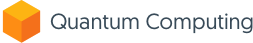## Answered Count by Post State

0

(click on this box to dismiss)Q&A for engineers, scientists, programmers, and computing professionals interested in quantum computing

``````SELECT
*,
CASE
ROUND(
(100.0 * NumPosts) / SUM(NumPosts) OVER (PARTITION BY HasAnswer),
2
)
ELSE 0
CASE
ROUND(
(100.0 * NumPosts) / SUM(NumPosts) OVER (PARTITION BY HasAnswer),
2
)
ELSE 0
ROUND(
(100.0 * NumPosts) / SUM(NumPosts) OVER (PARTITION BY State),
2
) AS PercentOfState,
CASE
WHEN Visible = 'Yes' THEN
ROUND(
(100.0 * NumPosts) / SUM(NumPosts) OVER (PARTITION BY Visible),
2
)
ELSE 0
END AS PercentOfVisible,
ROUND(
(100.0 * NumPosts) / SUM(NumPosts) OVER (),
2
) AS PercentAll
FROM (
SELECT
State,
Visible,
COUNT(*) AS NumPosts
FROM (
SELECT
Questions.Id,
CASE
WHEN COUNT(Answers.Id) >= 1 THEN 'Yes'
ELSE 'No'
CASE
WHEN Questions.DeletionDate IS NOT NULL THEN 'Deleted'
WHEN Questions.ClosedDate IS NOT NULL THEN 'Closed'
ELSE 'Open'
END AS State,
CASE
WHEN Questions.DeletionDate IS NULL THEN 'Yes'
ELSE 'No'
END AS Visible
FROM PostsWithDeleted AS Questions
LEFT JOIN PostsWithDeleted AS Answers ON (
)
WHERE Questions.PostTypeId = 1
GROUP BY Questions.Id, Questions.DeletionDate, Questions.ClosedDate
) AS QDataHold tight while we fetch your results